# Texas Go Math Grade 3 Lesson 9.2 Answer Key Multiplication Strategies with Multiples of 10

Refer to our Texas Go Math Grade 3 Answer Key Pdf to score good marks in the exams. Test yourself by practicing the problems from Texas Go Math Grade 3 Lesson 9.2 Answer Key Multiplication Strategies with Multiples of 10.

## Texas Go Math Grade 3 Lesson 9.2 Answer Key Multiplication Strategies with Multiples of 10

Essential Question
What strategies can you use to multiply with multiples of 10?

Unlock the Problem
You can use models and place value to multiply with multiples of 10.

• What is a product of 10 and the counting numbers 1, 2, 3, and so on?
A multiple of a number is the product of the number and a counting number.

Explanation:
Multiple of 10 and the counting number is going to be the product.
For example:
10 × 1 = 10.
10 × 2 = 20.
10 × 3 = 30.
And so on……

Activity
Model multiples of 10.
Materialsbase-ten blocks
Model the first nine multiples of 10.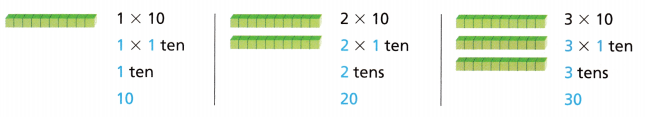What are the first nine multiples of 10?
10, 20, 30, __, ___, ___, ___, ___, ____
Best Care Veterinary Clinic offered free pet care classes for 5 days. Erin attended the pet care class for 30 minutes each day. How many minutes did Erin attend the class?

One Way
Use a number line.
5 × 30 = __
Think: 30 = 3 tens

Step 1
Complete the number line. Write the labels for the multiples of 10.

Step 2
Draw jumps on the number line to show 5 groups of 3 tens.So, Erin attended the pet care class for ___ minutes.

So, Erin attended the pet care class for 150 minutes.
5 × 30 = 150.

Explanation:
Number of days Best Care Veterinary Clinic offered free pet care classes = 5 days.
Time of Erin attended the pet care class each day = 30 minutes.
Number of minutes Erin attend the class = Number of days Best Care Veterinary Clinic offered free pet care classes × Time of Erin attended the pet care class each day
= 5 × 30
= (5 × 10) + (5 × 10) + (5 × 10)
= 50 + 50 + 50
= 100 + 50
= 150.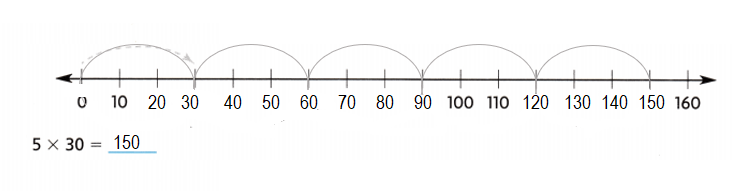Another Way
Use place value.So, 5 × 30 = ___

Think
5 × 30 = 5 × __ tens
= ___ tens = ___
5 × 30 = 150.

Explanation:
Using Place value:
5 × 30 = 5 × 3 tens(30)
= 15 tens = 15 × 10 = 150.

Math Talk
Mathematical processes
Explain why 5 × 30 has one zero in the product and 4 × 50 has two zeros in the product.
5 × 30 has one zero in the product and 4 × 50 has two zeros in the product because 5 is multiplied with 3 into 10  so product is 15 into 10  = 150 and 4 is multiplied with 5 into 10 so product is 20 into 10 = 200.

Explanation:
Product of 5 × 30:
= (5 × 10) + (5 × 10) + (5 × 10)
= 50 + 50 + 50
= 100 + 50
= 150.
Product of 4 × 50:
= (4 × 10) +  (4 × 10)  + (4 × 10)  + (4 × 10)  + (4 × 10)
= 40 + 40 + 40 + 40 + 40
= 80 + 40 + 40 + 40
= 120 + 40 + 40
= 160 + 40
= 200.

Share and Show
Use the number line to find the product.
Question 1.
3 × 40 = ___
Think: There are 3 jumps of 40.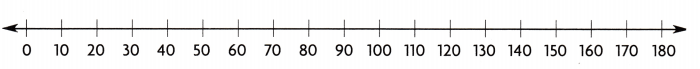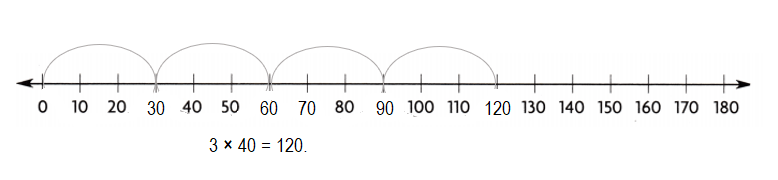Explanation:
3 × 40 = (3 × 10) + (3 × 10) + (3 × 10) + (3 × 10)
= 30 + 30 + 30 + 30
= 60 + 30 + 30
= 90 + 30
= 120.

Question 2.
8 × 20 = ___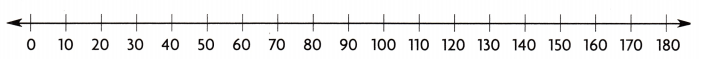Explanation:
8 × 20 = (8 × 10) + (8 × 10)
= 80 + 80
= 160.

Use place value to find the product.

Question 3.
3 × 70 = 3 × __ tens
= __ tens = ___
3 × 70 = 210.

Explanation:
3 × 70 = 3 × 7 tens
= 21 tens = 21 × 10 = 210.

Question 4.
50 × 2 = __ tens × 2
= __ tens = ___
50 × 2 = 100.

Explanation:
50 × 2 = 5 tens × 2
= 10 tens = 10 × 10 = 100.

Math Talk
Mathematical Processes
Will the product of 50 × 2 be the same as the product of 2 × 50? Explain.
Yes, the product of 50 × 2 be the same as the product of 2 × 50 will be the same by using distributive property where (a × b = b × a).

Explanation:
Product of :
50 × 2 = 100.
2 × 50 = 100.

Use the table for 5-7.
Question 5.
Representations The cost of a bottle of shampoo is $9. If the clinic sells their entire supply of shampoo, how much money will they receive?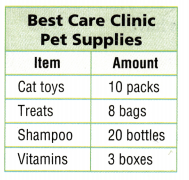Answer: Amount of money for the entire supply of shampoo received =$180.

Explanation:
Number of bottles of shampoo = 20.
Amount of money  for each bottle of shampoo = $9. Amount of money for the entire supply of shampoo received = Number of bottles of shampoo × Amount of each bottle of shampoo = 20 ×$9
= (10 × $9) + (10 ×$9)
= $90 +$90
= \$180.

Question 6.
What’s the Question? Each bag of treats has 30 treats. The answer is 240.
Question: How bags of treats the clinic sold.

Explanation:
Number of treats in each bag of treats = 30.
Division:
Answer given ÷ Number of treats in each bag of treats
= 240 ÷ 30
= (24 × 10) ÷ (3 × 10)
= 24 ÷ 3
= 8.

Question 7.
Multi-Step There are 4 bottles of vitamins in each box of vitamins. Each bottle of vitamins has 20 vitamins. If the clinic wants to have a supply of 400 vitamins, how many more boxes should they order?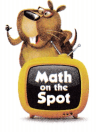Number of more boxes they should order = 5.

Explanation:
Number of bottles of vitamins in each box of vitamins = 4.
Number of vitamins each bottle of vitamins has = 20.
Number of vitamins in the boxes of vitamins = Number of bottles of vitamins in each box of vitamins × Number of vitamins each bottle of vitamins has
= 4 × 20
= (4 × 10) + (4 × 10)
= 40 + 40
= 80.
Total number of vitamins the clinic supplies = 400.
Number of more boxes they should order = Total number of vitamins the clinic supplies ÷ Number of vitamins in the boxes of vitamins
= 400 ÷ 80
= (4 × 10 × 10) ÷ (8 ×10)
= (4 × 10) ÷ 8
= 5.

Question 8.
Multi-Step Hiromi needs to set up 155 chairs for people to attend pet care classes. So far she has set up 6 rows with 20 chairs in each row. How many more chairs does Hiromi need to set up?
Number of chairs more she needs to set up = 35.

Explanation:
Total number of chairs for people to attend pet care classes Hiromi needs to set up = 155.
Number of rows she set up = 6.
Number of chairs in each row she set up = 20.
Number of chairs she set up till now = Number of rows she set up × Number of chairs in each row she set up
= 6 × 20
= (6 × 10) + (6 × 10)
= 60 + 60
= 120.
Number of chairs more she needs to set up = Total number of chairs for people to attend pet care classes Hiromi needs to set up – Number of chairs she set up till now
= 155 – 120
= 35.

Question 9.
Multi-Step Last week, Dr. Newman examined the paws of 30 dogs at her clinic. She examined the paws of 20 cats. What is the total number of paws Dr. Newman examined last week?
Total number of paws Dr. Newman examined last week = 50.

Explanation:
Number of paws of dogs Dr. Newman examined at her clinic last week = 30.
Number of paws of cats Dr. Newman examined at her clinic last week = 20.
Total number of paws Dr. Newman examined last week = Number of paws of dogs Dr. Newman examined at her clinic last week + Number of paws of cats Dr. Newman examined at her clinic last week
= 30 + 20
= 10(3 + 2)
= 10 × 5
= 50.

Fill in the bubble for the correct answer choice.
Question 10.
Orca whales have 50 teeth. How many teeth do 6 orcas have?
(A) 560
(B) 30
(C) 300
(D) 56
Total number of teeth Orca whales have =  300.
(C) 300.

Explanation:
Number of teeth Orca whales have = 50.
Number of Orca whales = 6.
Total number of teeth Orca whales have =  Number of teeth Orca whales have × Number of Orca whales
= 50 × 6
= (10 × 6) + (10 × 6) + (10 × 6) + (10 × 6) + (10 × 6)
= 60 + 60 + 60 + 60 + 60
= 120 + 60 + 60 + 60
= 180 + 60 + 60
= 240 + 60
= 300.

Question 11.
Apply A song mentions 10 drummers drumming. Singers repeat that part 3 times. 1f they are singing about different drummers in each part, how many drummers are they singing about in all?
(A) 13
(B) 30
(C) 310
(D) 130
Number of drummers are they singing about in all = 30.
(B) 30.

Explanation:
Number of drummers drumming a song mentions = 10.
Number of times Singers repeats that part = 3.
Number of drummers are they singing about in all = Number of drummers drumming a song mentions × Number of times Singers repeats that part
= 10 × 3
= 30.

Question 12.
Multi-Step The U.S. flag has 50 stars. One box has 2 U.S. flags in it. How many stars are in 8 boxes?
(A) 800
(B) 40
(C) 400
(D) 100
Number of stars in 8 boxes of U.S. flag = 800.
(A) 800.

Explanation:
Number of stars the U.S. flag has = 50.
Number of flags one box has =2.
Number of stars in U.S. flag box has = Number of stars the U.S. flag has × Number of flags one box has
= 50 × 2
= (10 × 2) + (10 × 2) + (10 × 2) + (10 × 2) + (10 × 2)
= 20 + 20 + 20 + 20 + 20
= 40 + 20 + 20 + 20
= 60 + 20 + 20
= 80 + 20
= 100.
Number of stars in 8 boxes of U.S. flag = 8 × Number of stars in U.S. flag box has
= 8 × 100
= 800.

Texas Test Prep
Question 13.
There are 8 rows of chairs set up in the gym for the career day program. If there are 30 chairs in each row, how many chairs are there?
(A) 38
(B) 240
(C) 80
(D) 22
Total number of chairs are there = 240.
(B) 240.

Explanation:
Number of rows of chairs set up in the gym for the career day program = 8.
Number of chairs in each row = 30.
Total number of chairs are there = Number of rows of chairs set up in the gym for the career day program  × Number of chairs in each row
= 8 × 30
= (8 × 10) + (8 × 10) + (8 × 10)
= 80 + 80 + 80
= 160 + 80
= 240.

### Texas Go Math Grade 3 Lesson 9.2 Homework and Practice Answer Key

Use the number line to find the product.

Question 1.
5 × 30 = ___Explanation:
5 × 30 = (5 × 10) + (5 × 10) + (5 × 10)
= 50 + 50 + 50
= 100 + 50
= 150.

Question 2.
7 × 20 = ___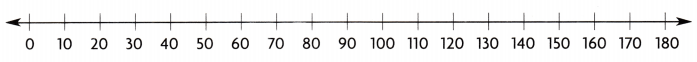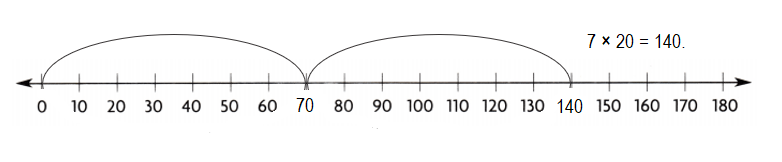Explanation:
7 × 20 = (7 × 10) + (7 × 10)
= 70 + 70
= 140.

Use place value to find the product.

Question 3.
4 × 60 = 4 × __ tens
= ___ tens = ____
4 × 60 = 240.

Explanation:
4 × 60 = 4 × 6 tens
= 240 tens = 24 × 10 = 240.

Question 4.
30 × 9 = ___ tens × 9
= ___ tens = ___ tens
30 × 9 = 270.

Explanation:
30 × 9 = 3 tens × 9
= 27 tens = 27 × 10 = 270.

Problem Solving
Use the table for 5-7.
Question 5.
At the sports store, there are 70 bike helmets in each crate. If the store sells all the crates, how many bike helmets Bike helmets does the store sell?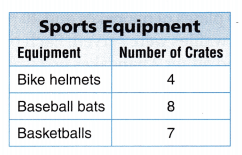Total number of Bike helmets does the store sell = 280.

Explanation:
Number of bike helmets in each crate = 70.
Number of crates sports equipment has of bike helmets = 4.
Total number of Bike helmets does the store sell = Number of bike helmets in each crate × Number of crates sports equipment has of bike helmets
= 70 × 4
= 280.

Question 6.
There are 30 basketballs in each crate. How many basketballs are there in all?
Number of basketballs are there in all = 210.

Explanation:
Number of basketballs in each crate = 30.
Number of crates of basketballs = 7.
Number of basketballs are there in all = Number of basketballs in each crate × Number of crates of basketballs
= 30 × 7
= (10 × 7) + (10 × 7) + (10 × 7)
= 70 + 70 + 70
= 140 + 70
= 210.

Question 7.
Each crate of baseball bats contains 30 bats. If the store sells 2 crates of bats, how many bats are left?
Number of bats are left = 180.

Explanation:
Number of bats each crate of baseball bats contains = 30.
Number of crates of bats the store sells = 2.
Number of crates of bats the store has = 8.
Total number of bats the store has = Number of bats each crate of baseball bats contains × Number of crates of bats the store has
= 30 × 8
= (10 × 8) + (10 × 8) + (10 × 8)
= 80 + 80 + 80
= 160 + 80
= 240.
Total number of bats the store sells = Number of bats each crate of baseball bats contains × Number of crates of bats the store sells
= 30 × 2
= (10 × 2) + (10 × 2) + (10 × 2)
= 20 + 20 + 20
= 40 + 20
= 60.
Number of bats are left = Total number of bats the store has – Total number of bats the store sells
= 240 – 60
= 10(24 – 6)
= 10 × 18
= 180.

Texas Test Math
Lesson Check
Question 8.
A school cafeteria has 30 tables. Each table seats 6 students. How many students can be seated?
(A) 120
(B) 150
(C) 180
(D) 306
Number of students can be seated = 180.
(C) 180.

Explanation:
Number of tables a school cafeteria has = 30.
Number of seats for students in each table = 6.
Number of students can be seated = Number of tables a school cafeteria has × Number of seats for students in each table
= 30 × 6
= (10 × 6) + (10 × 6) + (10 × 6)
= 60 + 60 + 60
= 120 + 60
= 180.

Question 9.
The cafeteria serves 50 bowls of fruit. Each bowl has 8 pieces of fruit. How many pieces of fruit were served?
(A) 508
(B) 400
(C) 450
(D) 40
Number of pieces of fruit were served = 400.
(B) 400.

Explanation:
Number of bowls of fruits the cafeteria serves = 50.
Number of pieces of fruit each bowl has = 8.
Number of pieces of fruit were served = Number of bowls of fruits the cafeteria serves × Number of pieces of fruit each bowl has
= 50 × 8
= (10 × 8) + (10 × 8) + (10 × 8)+ (10 × 8)+ (10 × 8)
= 80 + 80 + 80 + 80 + 80
= 160 + 80 + 80 + 80
= 240 + 80 + 80
= 320 + 80
= 400.

Question 10.
In the gym, there are 7 rows of bleacher seats. Each row holds 40 people. How many people can sit in the bleacher seats?
(A) 240
(B) 210
(C) 407
(D) 280
Number of people can sit in the bleacher seats = 280.
(D) 280.

Explanation:
Number of rows of bleacher seats in the gym = 7.
Number of people each row holds = 40.
Number of people can sit in the bleacher seats = Number of rows of bleacher seats in the gym × Number of people each row holds
= 7 × 40
= (7 × 10) + (7 × 10) + (7 × 10) + (7 × 10)
= 70 + 70 + 70 + 70
= 140 + 70 + 70
= 210 + 70
= 280.

Question 11.
A marching band concert has a total of 9 bands. Each band has 50 students. How many students are in the marching bands?
(A) 480
(B) 540
(C) 409
(D) 450
Number of students are in the marching bands = 450.
(D) 450.

Explanation:
Total number of bands A marching band concert has = 9.
Number of students each band has = 50.
Number of students are in the marching bands = Total number of bands A marching band concert has × Number of students each band has
= 9 × 50
= (9 × 10) + (9 × 10) + (9 × 10)+ (9 × 10) + (9 × 10)
= 90 + 90 + 90 + 90 + 90 + 90
= 180 + 90 + 90 + 90
= 270 + 90 + 90 +
= 360 + 90
= 450.

Question 12.
Multi-Step At the school brunch, 20 plates of pancakes and 40 plates of waffles were served. Each plate had either 2 pancakes or 2 waffles. How many waffles and pancakes were served?
(A) 120
(B) 200
(C) 60
(D) 280
Number of waffles and pancakes were served = 120.
(A) 120.

Explanation:
Number of plates of pancakes were served = 20.
Number of pancakes each plate had = 2.
Total number of pancakes served = Number of plates of pancakes were served  × Number of pancakes each plate had
= 20 × 2
= (10× 2) + (10× 2)
= 20 + 20
= 40.
Number of plates of waffles were served = 40.
Number of waffles each plate had = 2.
Total number of waffles served = Number of plates of waffles were served × Number of waffles each plate had
= 40 × 2
= (10 × 2) + (10 × 2) + (10 × 2) + (10 × 2)
= 20 + 20 + 20 + 20
= 40 + 20 + 20
= 60 + 20
= 80.
Number of waffles and pancakes were served = Total number of pancakes served + Total number of waffles served
= 40 + 80
= 10 (4 + 8)
= 10 × 12
= 120.

Question 13.
Multi-Step At the football game, there are 6 cartons of apple juice and 8 cartons of grape juice. Each carton holds 40 bottles of juice. How many bottles of juice are there?
(A) 320
(B) 560
(C) 650
(D) 480
Total number of bottles of juice are there = 560.
(B) 560.

Explanation:
Number of cartons of apple juice at the football game = 6.
Number of cartons of grape juice at the football game = 8.
Number of bottles of juice each carton holds = 40.
Total number of cartons of apple juice = Number of cartons of apple juice at the football game × Number of bottles of juice each carton holds
= 6 × 40
= (6 × 10) + (6 × 10) + (6 × 10)  + (6 × 10)
= 60 + 60 + 60 + 60
= 120 + 60 + 60
= 180 + 60
= 240.
Total number of cartons of grape juice = Number of cartons of grape juice at the football game × Number of bottles of juice each carton holds
= 8 × 40
= (8 × 10) + (8 × 10) + (8 × 10)  + (8 × 10)
= 80 + 80 + 80 + 80
= 160 + 80 + 80
= 240 + 80
= 320.
Total number of bottles of juice are there = Total number of cartons of apple juice + Total number of cartons of grape juice
= 240 + 320
= 10 (24 + 32)
= 10 × 56
= 560.

Scroll to Top